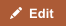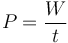In physics, energy is defined as the amount of work that can be performed by force, whereas power is defined as the rate at which work is performed.

## Comparison chart

Energy versus Power comparison chartEnergyPower
Definition Energy is the capacity to do work. Energy is power integrated over time. Power is the rate at which work is done, or energy is transmitted.
Unit joules = watt-seconds or joule = Newton-meter watt = joules/second
Common symbol(s) W P
Example I left a 60W light bulb on for 30 days, which raised my electric bill by 43.2 kWh (kilowatt-hours). My car's battery can provide 500 amps at 12 volts, which equals 6kW of power.

## Different Forms of Power and Energy

There are different forms of energy. These include kinetic, potential, thermal, gravitational, electromagnetic, sound, light and elastic. The form of energy is dependent on the frame of reference, and can be transformed into other forms. For instance potential energy is dependent on the position of the object, whereas kinetic energy is the energy required to accelerate an object to a particular speed, and so on.

Different forms of power could be electric power, which is the rate at which electrical energy is transferred by a circuit, human power, and optical power.

## Transformation of Energy

Various devices can be used to convert one form of energy into another. For instance, a battery converts chemical to electric energy, chemical explosion converts chemical energy in to kinetic and thermal energy and so on. Power cannot be converted or transformed.

## Measuring Energy vs. Power

Although it is not possible to directly measure energy, the work done can be defined and measured. The methods involves using a calorimeter, which measures the heat absorbed or released in chemical reactions or physical changes, thermometer, which measures temperature or bolometer that is employed to measure the intensity of radiation. Energy generated can be stored whereas power cannot.

Since power is energy per unit of time, in theory it can be calculated after measuring the energy used per second. When calculating the real power consumption of an electrical device, it is essential to measure the voltage applied and the current consumed, taking into account the power that is dissipated in the circuit.

## Difference in Units

Energy is usually measured in Joules, the other units include ergs and calories. Power is measured in Watts, which is Joules per second, or ergs per second. Machine are usually described by its power rating, the higher the number the more powerful the machine.

## Equation

Power is an energy per unit of time. As a rate of change of work done or the energy of a subsystem, power is:$P = \frac{W}{t}\,$ where P is power, W is work and t is time.